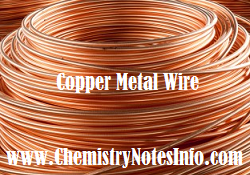# Chemistry MCQ

## Chemistry General Knowledge as Chemistry Multiple Choice Question Answer

### Q1. After some time period electrochemical cell stop working due to

• Direction of rate of reaction get reverse
• One Electrode get completely consumed
• Both Electrodes get completely consumed
• Electrode potential of the both electrodes get equalizes
Ans of Chemistry GK MCQ Question Q1: Electrode potential of the both electrodes get equalizes

### Q2. _______ is used to produce artificial rain

• Carbon Monooxide
• Copper Oxide
• Silver Iodide
• Silver Nitrate
Ans of Chemistry GK MCQ Question Q2: Silver Iodide

### Q3. Zero Group elements are

• Unreactive Non-metals
• Reactive Metals
• Reactive Non-metals
• Unreactive Metals
Ans of Chemistry GK MCQ Question Q3: Unreactive Non-metals

### Q4. Reaction between the chlorine and methane in the presence of diffused sunlight is

• Polymerization
• Oxidation
• Substitution
• Reduction
Ans of Chemistry GK MCQ Question Q4: Substitution

### Q5. Copper is recovered from the solution of copper sulphate, with the help of• Na
• Hg
• Ag
• Fe
Ans of Chemistry GK MCQ Question Q5: Fe

### Q6. Oxidation reaction represents

• Electrons are lost
• Electrons are gained
• Protons are lost
• Protons are gained
Ans of Chemistry GK MCQ Question Q6: Electrons are lost

### Q7. _______ acid is also known as oil of vitriol

• Phosphoric acid
• Hydrochloric acid
• Sulphuric acid
• Aquaregia
Ans of Chemistry GK MCQ Question Q7: Sulfuric acid

### Q8. Proton have ___ charge and Electron have ___ charge

• -1,  +1
• -1,  -2
• +1,  -1
• +2,  -2
Ans of Chemistry GK MCQ Question Q8: +1,  -1

### Q9. ______ aluminum alloy is used in manufacturing of magnets

• Alnico
• Aluminum bronze
• Y-Alloy
• None
Ans of Chemistry GK MCQ Question Q9: Alnico

### Q10. Number of electrons in the d-orbital of Fe+2 (Z=26) is not equal to

• Number of electrons in p-orbital of Ne (Z=10)
• Number of electrons in d-orbital of Fe (Z=26)
• Number of electrons in s-orbital of Mg (Z=12)
• Number of electrons in p-orbital of Cl (Z=17)
Ans of Chemistry GK MCQ Question Q10: Number of electrons in p-orbital of Cl (Z=17)

We publish some chemistry general knowledge question-answer (Chemistry MCQs) to increase your chemical science GK.